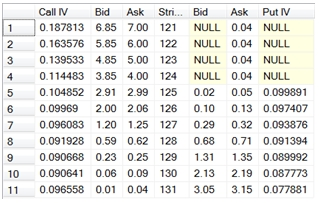# SQL Server Binomial Tree function for European option implied volatility

BinomialEuroIV

Updated: 04 Sep 2012

Use BinomialEuroIV to calculate the implied volatility of a European option using the Binomial Tree option pricing formula.
Syntax
SELECT [wctOptions].[wct].[BinomialEuroIV] (
<@CallPut, nvarchar(4000),>
,<@AssetPrice, float,>
,<@StrikePrice, float,>
,<@TimeToMaturity, float,>
,<@RiskFreeRate, float,>
,<@DividendRate, float,>
,<@Price, float,>
,<@NSteps, int,>)
Arguments
@CallPut
identifies the option as being a call ('C') or a put ('P'). @CallPut is an expression of type nvarchar or of a type that can be implicitly converted to nvarchar.
@AssetPrice
the price of the underlying asset. @AssetPrice is an expression of type float or of a type that can be implicitly converted to float.
@StrikePrice
the exercise price of the option. @StrikePrice is an expression of type float or of a type that can be implicitly converted to float.
@TimeToMaturity
the time to expiration of the option, expressed in years. @TimeToMaturity is an expression of type float or of a type that can be implicitly converted to float.
@RiskFreeRate
the annualized, continuously compounded risk-free rate of return over the life of the option. @RiskFreeRate is an expression of type float or of a type that can be implicitly converted to float.
@DividendRate
the annualized, continuously compounded dividend rate over the life of the option. For currency options, @Div should be the foreign risk-free interest rate. @DividendRate is an expression of type float or of a type that can be implicitly converted to float.
@Price
the observed price of the option. @Price is an expression of type float or of a type that can be implicitly converted to float.

@nSteps
the number of steps in the binomial tree. @nSteps is an expression of type int or of a type that can be implicitly converted to int.
Return Type
float
Remarks
·         @TimeToMaturity must be greater than zero (@TimeToMaturity > 0).
·         @AssetPrice must be greater than zero (@AssetPrice > 0).
·         @StrikePrice must be greater than zero (@StrikePrice > 0).
·         @nSteps must be greater than 1 (@nSteps > 1)
·         If @DividendRate is NULL an error will be returned
·         If @RiskFreeRate is NULL an error will be returned
·         To calculate the price or Greeks of a European option use BlackScholesMerton or BinomialEuro.
·         To calculate the implied volatility of a European option using the Black-Scholes-Merton method, use BlackScholesMertonIV.
·         To calculate the implied volatility on American options use BjerksundStenslandIV or.
Example
Calculate the price for a call option on 2012-09-04, expiring on 2012-12-15, with a current asset price of 99.5, a strike price of 100 and a volatility of 20%. The observed price is 4.1547 and the dividend rate is 0.5%. The number of steps is 100.
SELECT ROUND(wct.BinomialEuroIV(
'C'         --PutCall
,99.5       --Asset Price
,100        --Strike Price
,datediff(d,'2012-09-04','2012-12-15') / 365.0000     --Time-to-expiry
,.02        --Risk Free Rate
,.005       --Dividend Rate
,4.1547           --Price
,100        --Number of Steps
), 5) as Volatility
This produces the following result.
Volatility
----------------------
0.2

In the following SELECT, we collect the implied volatility for a series of strike prices on EUR/USD option which expire in 7 days time. The option price is the midpoint of the bid and ask and the 7 day EURIBOR rate is 0.0335700% and the 7 day LIBOR rate is 0.1870000%.
SELECT
CASE
WHEN call_bid IS NULL THEN NULL
WHEN call_ask IS NULL THEN NULL
ELSE wct.BinomialEuroIV('C'
,127.91           --Asset price
,n.s              --Strike
,Cast(7 as float)/cast(365 as float) --Time
,0.000335699      --Continuously Compouned EURIBOR
,0.001869966      --Continuously Compouned LIBOR
,(call_bid + call_ask) / 2    --Price
,100              --Number of Steps
)
END as [Call IV]
,cast(n.call_bid as money) as Bid
,n.s as Strike
,cast(n.put_bid as money) as Bid
,CASE
WHEN put_bid IS NULL THEN NULL
WHEN put_ask IS NULL THEN NULL
ELSE wct.BinomialEuroIV('P'
,127.91           --Asset price
,n.s              --Strike
,Cast(7 as float)/cast(365 as float)      --Time
,0.000335699      --Continuously Compouned EURIBOR
,0.001869966      --Continuously Compouned LIBIBOR
,(put_bid + put_ask) / 2      --Price
,100              --Number of Steps
)
END as [Put IV]
FROM (
SELECT 6.85, 7.00, 121, NULL, .04 UNION ALL
SELECT 5.85, 6.00, 122, NULL, .04 UNION ALL
SELECT 4.85, 5.00, 123, NULL, .04 UNION ALL
SELECT 3.85, 4.00, 124, NULL, .04 UNION ALL
SELECT 2.91, 2.99, 125, .02, .05 UNION ALL
SELECT 2.00, 2.06, 126, .10, .13 UNION ALL
SELECT 1.20, 1.25, 127, .29, .32 UNION ALL
SELECT 0.59, 0.62, 128, .68, .71 UNION ALL
SELECT 0.23, 0.25, 129, 1.31, 1.35 UNION ALL
SELECT 0.06, 0.09, 130, 2.13, 2.19 UNION ALL
SELECT 0.01, .04, 131, 3.05, 3.15

This produces the following result.### SupportCopyright 2008-2023 Westclintech LLC         Privacy Policy        Terms of Service